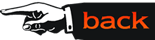## Mathematics of Change

This course is intended to introduce the essential ideas of Calculus in a motivated and meaningful way to future middle grade mathematics teachers. The course will expose these future teachers to the key aspects of the calculus, including such topics as slope, differentiation (rates of change), optimization, graphing, integration, area, and volume. Without compromising the integrity of the subject matter, we will primarily strive to develop an approach to Calculus that emphasizes how the subject relates to the real world and how it is connected to the mathematics they will be teaching in their classrooms. The course we intend to develop will be continually linked to existing (NSF funded) middle school curricula, with a variety of specific examples taken from these standards-based curricular materials. Such links will stimulate and motivate the students throughout the learning process, and the experience gained in the analysis of examples will help them to become better teachers.

To better illustrate our ideas, consider for a moment the question of optimization: Given a function, how does one find its maximum or minimum value? Since the underlying theme here is one of efficiency, techniques of optimization have widespread applications in fields as diverse as economics and finance, the applied sciences (physics, chemistry, etc.), art and architecture, and engineering. Consequently, it is crucial to provide students with an early introduction to the basic ideas of optimization. Middle school students are first exposed to these questions in geometric contexts, and while at this level they are not expected to develop a mathematically rigorous approach to the topic of optimization, they can still reach the correct conclusions via hands-on experiments. For example, in the series Math Thematics by McDougal Littell (Book 3, Section 6), students use graph paper to draw all possible rectangles with an area of 24 square units and whose length and width are whole numbers. For each such rectangle the perimeter is computed and the resulting data is plotted in a coordinate system with the width on the horizontal axis and the perimeter on the vertical axis. A number of questions can now be raised. (1) Does the graph show the perimeter of a rectangle with a width of 10 units? (2) Does the graph show the width of the rectangle with the least possible perimeter? (3) If one draws a curve to fit the points, can one estimate the perimeter of the rectangle with width 10 units? (4) Is there a greatest possible perimeter for a rectangle with area 24 square units? (5) Can one obtain an expression for the perimeters of the drawn rectangles in terms of the width?

This exercise leads naturally to questions in optimization, such as: Considering all rectangles with a fixed area, for which width is the perimeter maximized or minimized? In order to correctly facilitate the classroom exploration, teachers must possess a fairly deep understanding of the mathematical principles behind this problem and be able to employ multiple problem solving strategies in analyzing these questions. In connection to this, it is imperative that teachers have first-hand experiences in creative applications of the graphing calculator to such mathematics problems, and this in turn will empower them to effectively integrate this and other technologies into their classroom teaching.